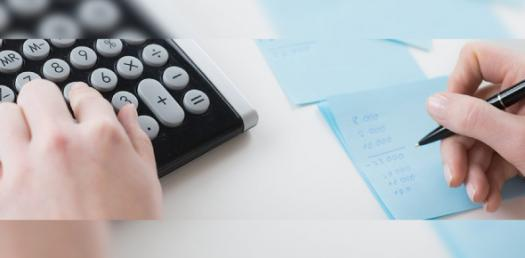# The Ultimate Accounting Quiz!

34 Questions | Total Attempts: 542SettingsWhat you see here is what is known as the Ultimate accounting quiz and most of the people who gunner over half the marks have what it takes to be certified in the field. Do you think you have what it takes to tackle it? Well, take up the quiz below and get to find out just how much you know about accounting!

Related Topics
• 1.
Which of these is not included as a separate item in the basic accounting equation?
• A.

Assets

• B.

Revenues

• C.

Liabilities

• D.

StockHolders Equity

• 2.
The accrual basis of accounting records revenues when they are:
• A.

Collected

• B.

Earned

• C.

Contracted

• D.

• 3.
The account format that displays debits, credits, balances, and headings.
• A.

General Journal

• B.

General Ledger

• C.

T-Account

• D.

Ledger Account

• 4.
Asset accounts have what type of balance?
• A.

Debit

• B.

Credit

• C.

Cotra

• D.

All of the Above

• 5.
Which account is not a liability account?
• A.

Accounts Payable

• B.

Accrued Expenses

• C.

Cash

• D.

Notes Payable

• 6.
Which account increases equity?
• A.

Expenses

• B.

Withdrawals

• C.

Treasury Stock

• D.

Revenues

• 7.
A contra asset account has what type of balance?
• A.

Debit

• B.

Credit

• C.

Contra

• D.

All the Above

• 8.
The debts, which are to be repaid within a short period (year or less) are known as
• A.

Current liabilities

• B.

Fixed liabilities

• C.

Contingent liabilities

• D.

All of the above

• 9.
The unfavorable balance of Profit and Loss account should be:
• A.

• B.

Subtracted from current assets

• C.

Subtracted from capital

• D.

Subtracted from liabilities

• 10.
The process of recording is done
• A.

Two times a year

• B.

Once a year

• C.

Frequently during the accounting period

• D.

At the end of a accounting period

• 11.
General journal is a book of _______ entries
• A.

First

• B.

Original

• C.

Secondary

• D.

Generic

• 12.
Goods returned by customer should be debited to which of the following accounts?
• A.

Sales income account

• B.

Sales account

• C.

Return inward account

• D.

Expenses account

• 13.
Discount allowed is
• A.

• B.

• C.

• D.

• 14.
_________ is the evidence that a transaction took place
• A.

Source documents

• B.

Ledger

• C.

Bonds

• D.

Journals

• 15.
Journals are also referred as
• A.

Book of entries

• B.

Book of original entries

• C.

T account

• D.

Books of economic event

• 16.
Which of the following accounts will be credited if a company purchases building for cash?
• A.

Capital account

• B.

Fixed assets account

• C.

Building account

• D.

Cash account

• 17.
Discount for quick repayment of debt is normally referred as
• A.

• B.

Prompt payment discount

• C.

Cash discount

• D.

Bulk discount

• 18.
The first step in accounting process is
• A.

Recording the transaction

• B.

Identifying the transaction

• C.

Posting the transaction

• D.

Preparing the source documents

• 19.
A chart of accounts generally start with which of the following types of accounts?
• A.

Assets accounts

• B.

Liability accounts

• C.

Cash accounts

• D.

Revenue accounts

• 20.
When it is certain that a debt won’t be recovered. Which of the following is correct?
• A.

Provision for bad debt is created

• B.

Account receivable is credited

• C.

• D.

Sales is debited

• 21.
The opening balance of “provision for doubtful debts account” is \$1000 whereas the closing balance of debtors account is 100,000. What amount of provision for doubtful debts should be charged to income statement using a 5% provision for doubtful debts for the current accounting period?
• A.

\$5000

• B.

\$4000

• C.

\$2000

• D.

\$1000

• 22.
The opening and closing balances of provision for doubtful debts account are \$1000 and \$2000 whereas bad debts are totaled \$200
• A.

\$1000 is to be deducted from total debtors balance in the balance sheet

• B.

\$2000 is to be subtracted from total debtors balance in the balance sheet

• C.

\$1000 is to be added to total debtors balance in the balance sheet

• D.

\$2000 is to be added to total debtors balance in the balance sheet

• 23.
Which of the following double entries is used to transfer “bad debts recovered” to income statement?
• A.

Bad debts recovered Debit and income statement Credit

• B.

Income statement Debit and bad debts recovered Credit

• C.

Cash Debit and bad debts recovered Credit

• D.

Bad debts recovered debit and cash Credit

• 24.
What does aged debtors analysis signify?
• A.

shows how long debts have been outstanding

• B.

How old the customers are

• C.

How long does a business take to repay the bank loans

• D.

Minimum number of old debtors

• 25.
According to a general rule of accounting, the older a debts is outstanding, the more likelihood that the debt will turn out to be a
• A.